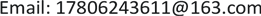1. 构建人口结构预测的主体模型

X a s ( t + 1 ) = X a s ( t ) H a s ( t ) + η 0 ∗ y a s ( t + 1 ) + ( − 1 ) a Z s ( t + 1 )

y a s ( t + 1 ) = ∑ b = 1 m y a b , s ( t + 1 ) = ∑ b = 1 m r a s ( t + 1 ) c a s ( t + 1 ) F a b ( t + 1 ) X 2 ( j , t + 1 ) ′

Z s ( t + 1 ) = γ a s ( t + 1 ) W s ( t + 1 ) ∗ X 1 s (t)

2. 实施二孩政策下的人口结构预测

2.1. 年龄结构预测

2.2. 城乡结构预测

2.3. 性别比例预测

3. 无二孩政策下人口结构的预测

4. 2030~2050年人口结构对经济发展的影响 [<xref ref-type="bibr" rid="hanspub.26134-ref4">4</xref>]4.1. 实施二孩政策下人均GDP预测

4.2. 无二孩政策下人均GDP预测

4.3. 结论分析